2018-06-18 20:25:13 weixin_40590063 阅读数 15464
• ###### MATLAB图像处理

MATLAB图像处理课程

4570 人正在学习 去看看 李立宗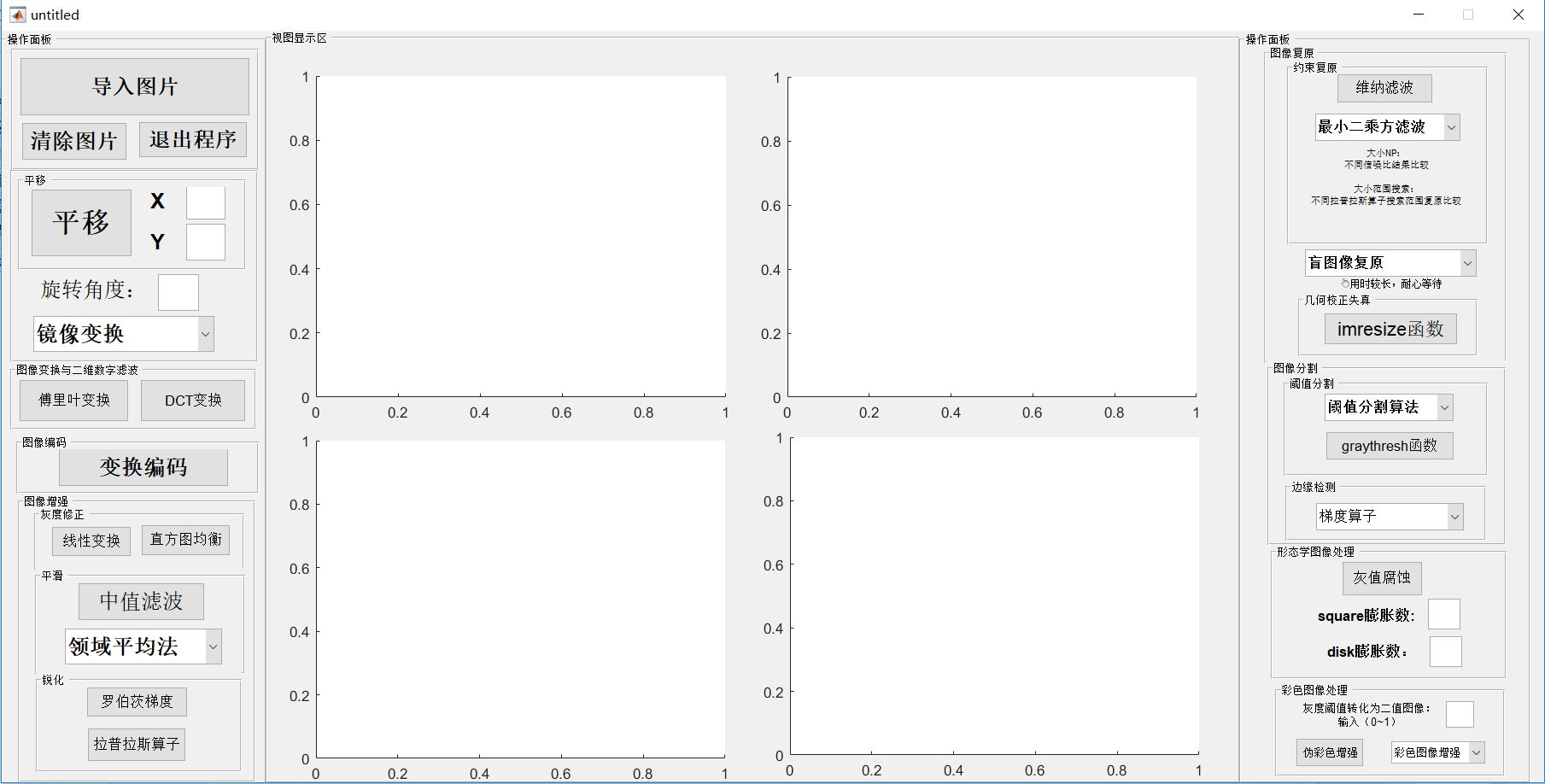# 正文内容

打开matlab后输入guide后弹出选择界面，新建一个GUI界面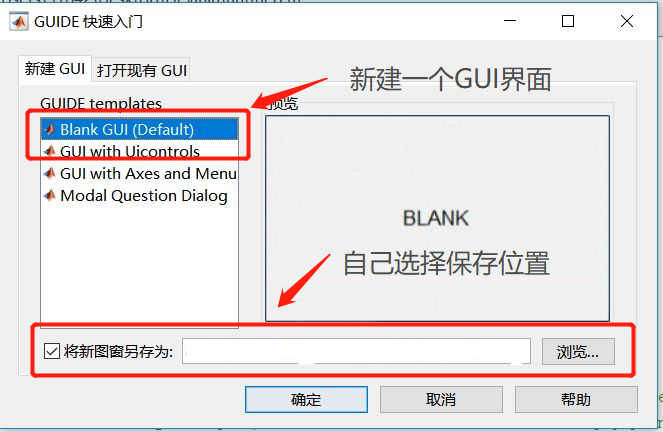（1）导入图片

```[file path]=uigetfile('*.bmp;*.jpg;*.png','请选择一幅图像');
if file==0 warndlg('您得输入一幅图像');
%警告对话框提示输入合法图像文件
else
axes(handles.axes1);
imshow(I);title('原图像');
handles.I=I;
end
%Update handles structure
guidata(hObject,handles);```

（2）清除图片和文本框内容

```%清除视图图片
cla(handles.axes2,'reset');  %handles.axes2为显示图片窗口，reset即清除
% 重置清空动态txt的文字
set(handles.edit1,'string','')；  %handles.edit1为要清除文字的文本框（双击文本框可以看见tag）```

（3）关闭程序

`close`

（4）平移（用文本框实现输入数字进行平移图像）

①先创建一个可编辑文本框（输入水平平移的位移），然后给他添加代码

```%获取text文本框的数字，竖直平移X的数值
global x;%定义一个x的全局变量
x=str2num(get(hObject,'String'));```

②先创建一个可编辑文本框（输入竖直平移的位移），然后给他添加代码

```%获取text文本框的数字，竖直平移Y的数值
global y;
y=str2num(get(hObject,'String'));```

③创建一个button，给他添加代码

```global y;
global x;
I=handles.I;
axes(handles.axes2);
se=translate(strel(1),[x y]);
j=imdilate(I,se);
axes(handles.axes2);
imshow(j);title('竖直平移后图像');```

在X和Y的文本框中输入数值，点击button后就能使图像平移了（如输入X：260；Y：90）（5）旋转

```%图像的旋转
I=handles.I;
a=str2num(get(hObject,'String'));
J1=imrotate(I, a);   %设置旋转角度，实现旋转并显示
axes(handles.axes2);
imshow(J1);title('旋转后图像');
guidata(hObject,handles);```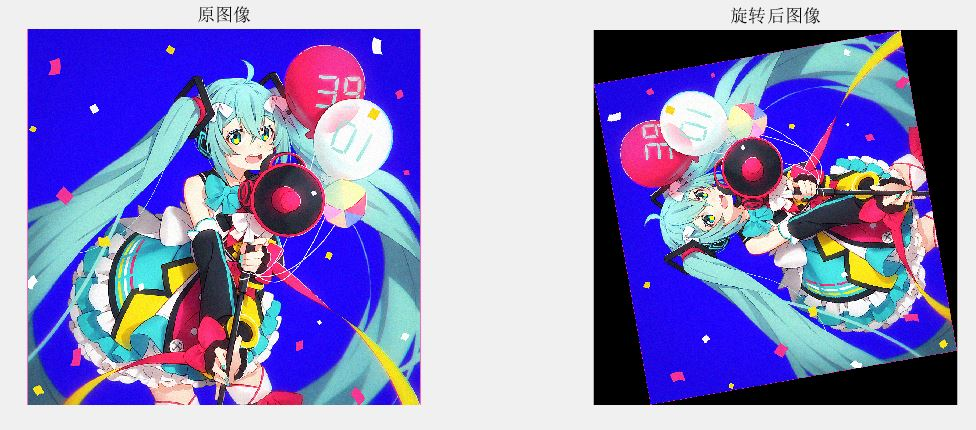（6）镜像

```%用下拉列表实现水平镜像，垂直镜像，水平垂直镜像
switch get(hObject,'value')   %实现下拉列表需要写改语法
case 1

case 2
I=handles.I;
J1=flipdim(I,2);%原图像的水平镜像
axes(handles.axes2);
imshow(J1);title('水平镜像');
guidata(hObject,handles);
case 3
I=handles.I;
J2=flipdim(I,1);%原图像的垂直镜像
axes(handles.axes2);
imshow(J2);title('垂直镜像');
guidata(hObject,handles);
case 4
I=handles.I;
J3=flipdim(I,1);%原图像的水平垂直镜像
J4=flipdim(J3,2);
axes(handles.axes2);
imshow(J4);title('水平垂直镜像');
guidata(hObject,handles);
end```（7）DCT变换

```%DCT变换（P58）
I=handles.I;
J=rgb2gray(I);%将图片转变为灰色图像
axes(handles.axes2);
imshow(J);title('原灰图像');
K=dct2(J);%对图像做DCT变换
axes(handles.axes3);
imshow(log(abs(K))+1,[0,10]);title('DCT变换结果');```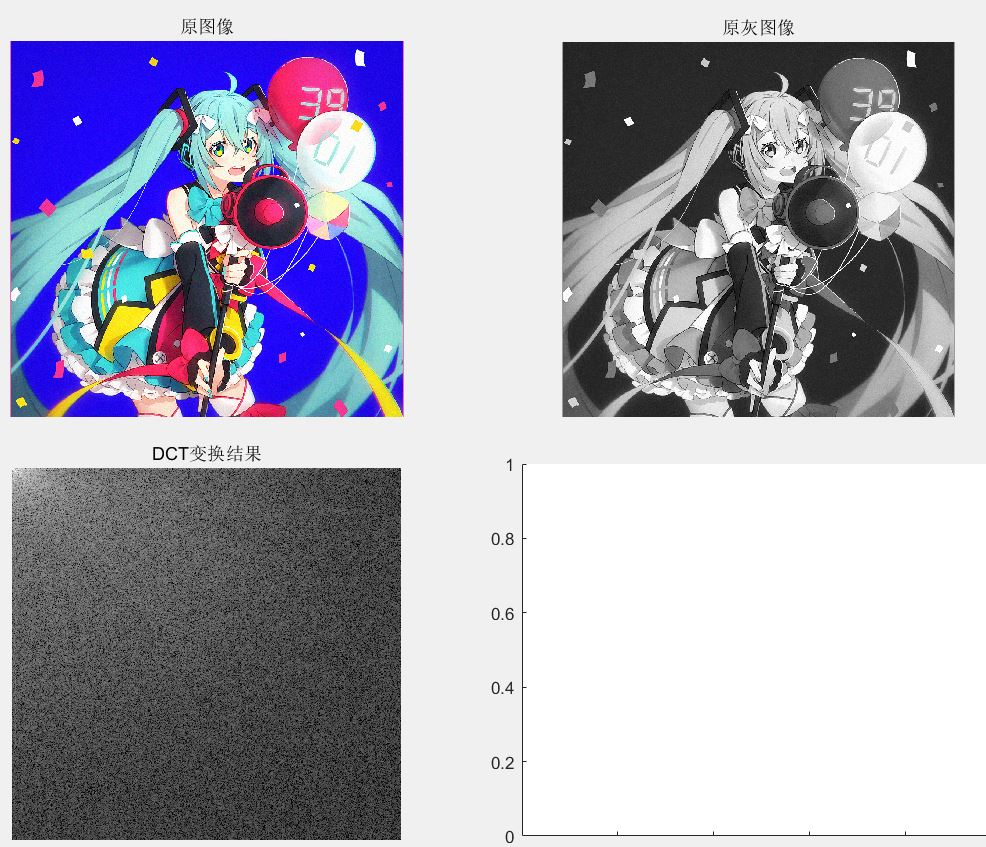（8）对受椒盐噪声污染的图像采用中值滤波去噪

```I=handles.I;
I=rgb2gray(I);%转化为灰度图像
J=imnoise(I,'salt & pepper',0.04);%对图像增加椒盐噪声，强度为0.04
axes(handles.axes2);    %显示在axex2框中
imshow(J); title('受椒盐噪声污染图片');
K=medfilt2(J);          %二维中值滤波
axes(handles.axes3);
imshow(K);title('二维中值滤波处理后的图片');```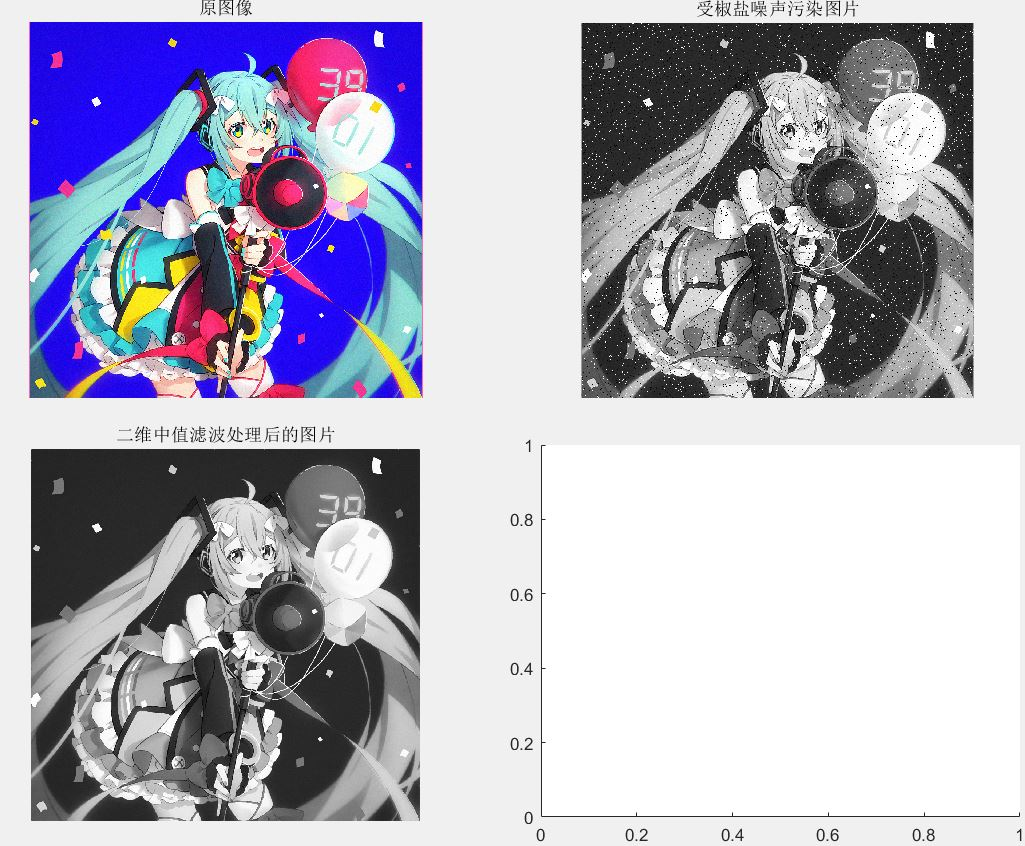（9）彩色图像增强（分别使用RGB和HSV方法）——用下拉列表实现

```switch get(hObject,'value')
case 1

case 2
RGB=handles.I;
R=RGB(:,:,1);
G=RGB(:,:,2);
B=RGB(:,:,3);
R1=histeq(R);
G1=histeq(G);
B1=histeq(B);
RGB1=cat(3,R1,G1,B1);
axes(handles.axes2);    %显示在axes2框中
imshow(RGB1);title('RGB增强');
case 3
RGB=handles.I;
R=RGB(:,:,1);
G=RGB(:,:,2);
B=RGB(:,:,3);
R1=histeq(R);
G1=histeq(G);
B1=histeq(B);
RGB1=cat(3,R1,G1,B1);
[H,S,V]=rgb2hsv(R,G,B);
V=histeq(V);
[R2,G2,B2]=hsv2rgb(H,S,V);
RGB2=cat(3,R2,G2,B2);
axes(handles.axes2);    %显示在axes2框中
imshow(RGB2,[]);title('HSV增强');
C=double(RGB1)./255-RGB2;
axes(handles.axes3);    %显示在axes3框中
imshow(C);title('差值图像');
end```

RGB增强: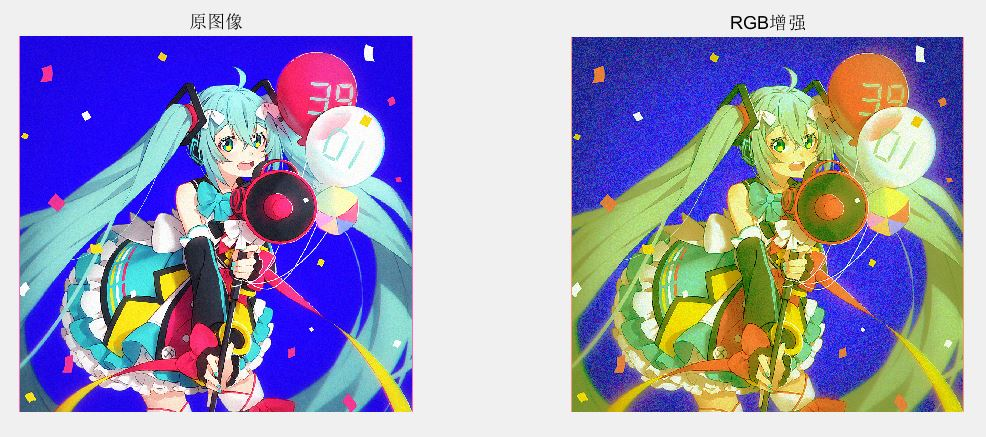HVS增强：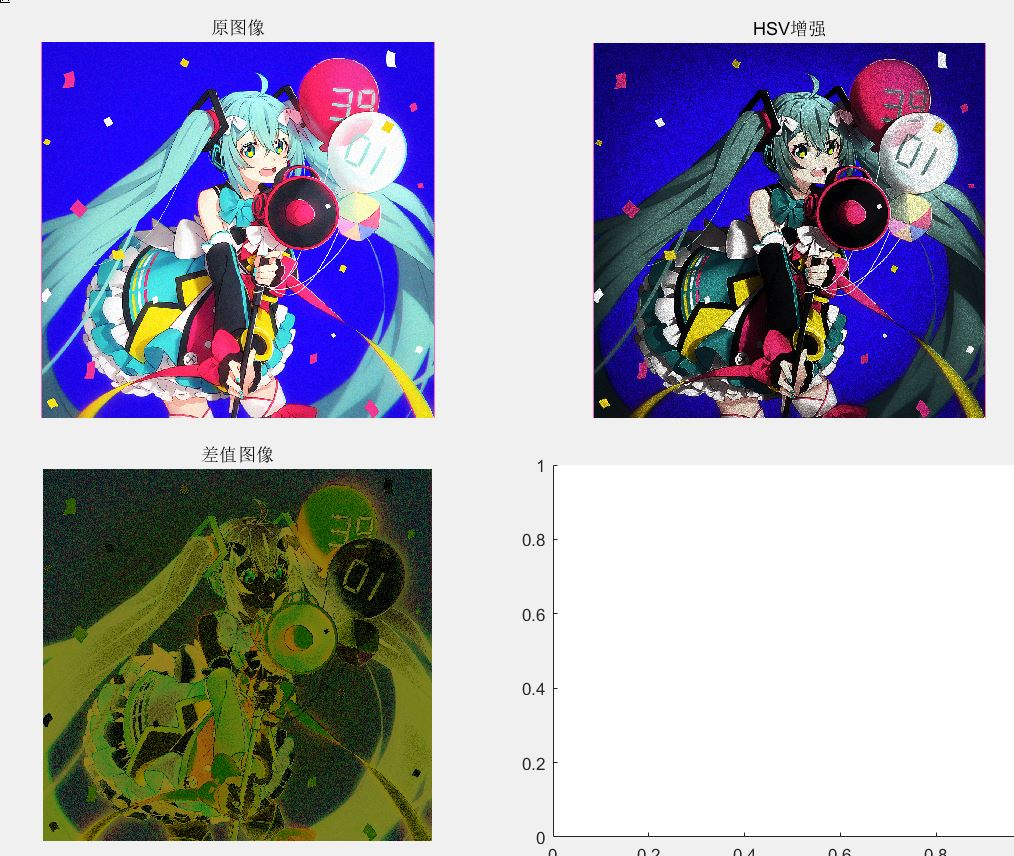（10）彩色图像锐化

```I=handles.I;
fb=tofloat(I);  	%将图像转化为浮点型
lapmask=[1 1 1;1 -8 1;1 1 1]; 	%拉普拉斯滤波模板
axes(handles.axes2);
imshow(fen);title('拉普拉斯锐化');
function [out,revertclass] = tofloat(inputimage)
%Copy the book of Gonzales
identify = @(x) x;
tosingle = @im2single;
table = {'uint8',tosingle,@im2uint8
'uint16',tosingle,@im2uint16
'logical',tosingle,@logical
'double',identify,identify
'single',identify,identify};
classIndex = find(strcmp(class(inputimage),table(:,1)));
if isempty(classIndex)
error('不支持的图像类型');
end
out = table{classIndex,2}(inputimage);
revertclass = table{classIndex,3};
```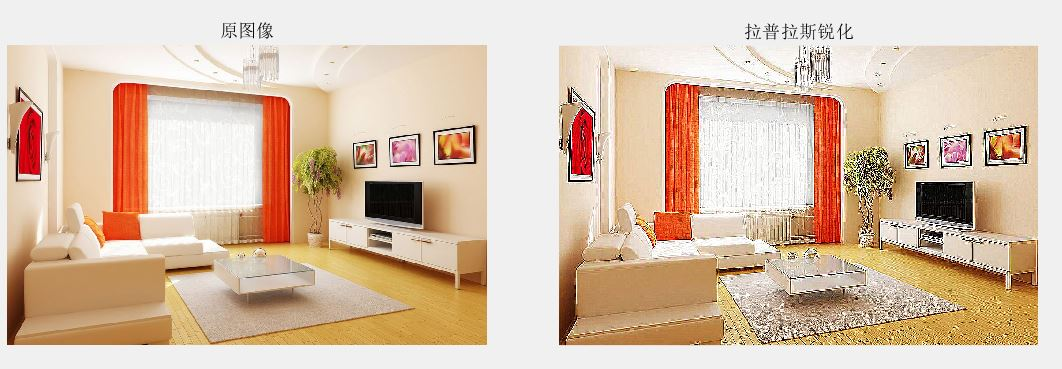（10）彩色图像复原

```%维纳滤波复原图像
I=handles.I;
len=28;
theta=14;
PSF=fspecial('motion',len,theta);
blurred=imfilter(I,PSF,'circular','conv');%读入无噪声模糊图像，并命名blurred
len=28;
theta=14;
wnrl=deconvwnr(blurred,PSF,0.04);%维纳滤波复原图像
axes(handles.axes2);
imshow(blurred);title('由运动形成模糊图像');%显示模糊图像
axes(handles.axes3);
imshow(wnrl);title('维纳滤波复原图像');%显示复原图像```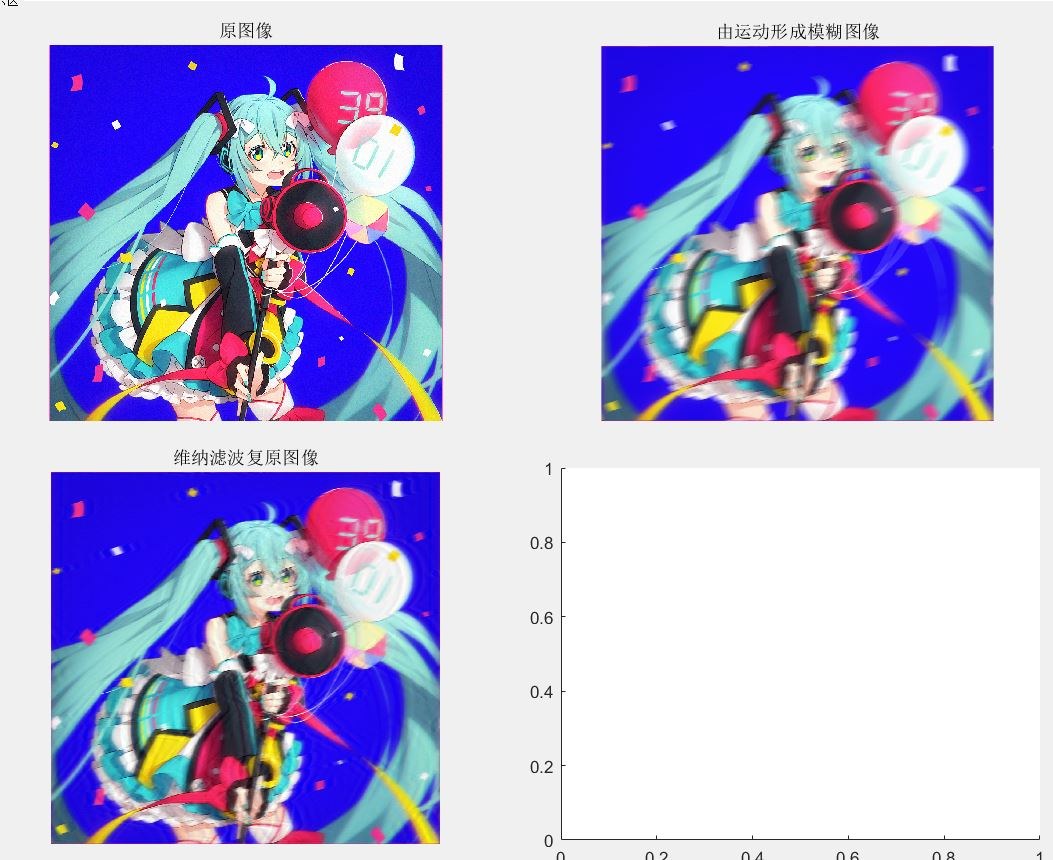（11）square膨胀

```%用square结构元素膨胀图片（用text文本框比较好）
a=str2num(get(hObject,'String'));
I=handles.I;
se1=strel('square',a);
I1=imerode(I,se1);
axes(handles.axes2);    %显示在axes2框中
imshow(I1);title('用square结构元素膨胀图片');```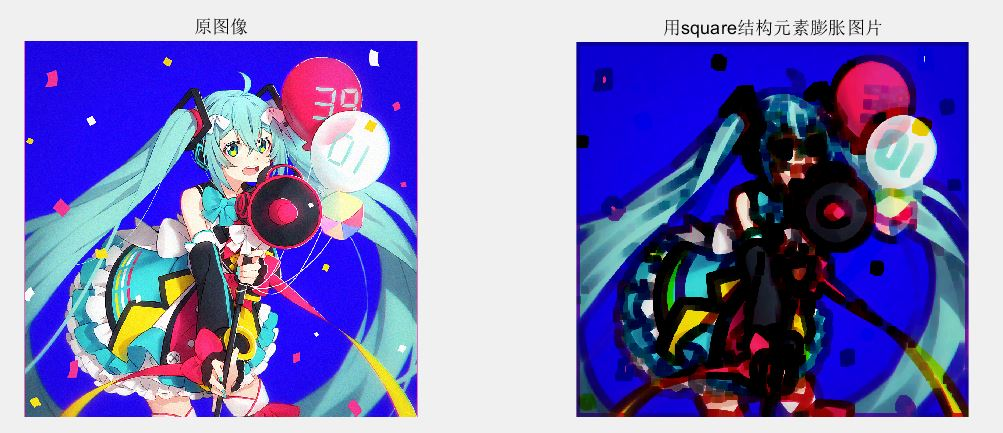这里输入了20的数值

（12）边缘检测（使用canny算子）

```I=handles.I;
I=rgb2gray(I);
BW5=edge(I,'canny');%进行canny算子边缘检测，门限值采用默认值
axes(handles.axes2);    %显示在axes2框中
imshow(BW5,[]);title('canny算子');```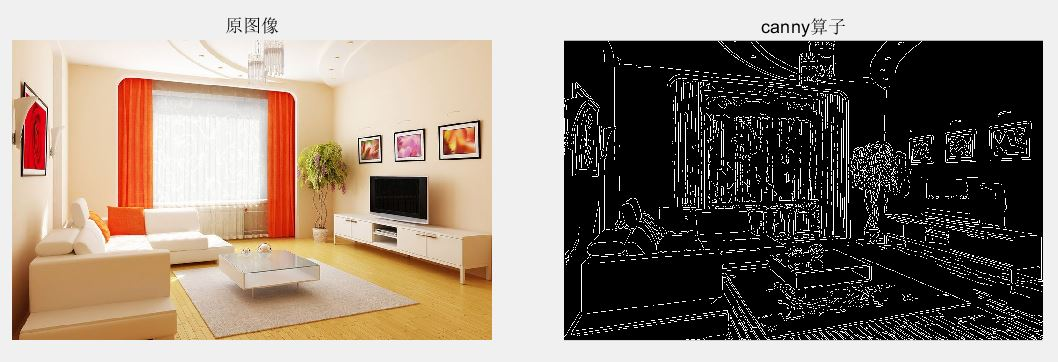2016-04-19 08:55:14 lfw2016 阅读数 17639
• ###### MATLAB图像处理

MATLAB图像处理课程

4570 人正在学习 去看看 李立宗

## 图像的邻域运算：

### 邻域运算主要作用是对图像进行空域滤波，比如平滑，中值滤波以及边缘检测等，此外，邻域运算还可以对结构图像进行细化。                        平滑： 平滑的目的是消除或尽量减少噪声，改善图像的质量。在matlab中用imfilter函数进行平滑操作，B  = imfilter(A, h); 其中A为原图像，h表示卷积核。                 A  = imread('1.jpg');               h = ones(3,3)/5;              %制定卷积核               h(1,1) = 0;               h (1,3) =0;               h(3,1) = 0;               h(1,3) = 0;              B  = imfilter(A,h);               %平滑处理              figure(1);             imshow(B);    中值滤波：               中值滤波用一个含有奇数点的滑动窗口，将邻域的像素按灰度级排序，取其中间值为输出像素。它的优点在于能够在抑制随机噪声的同时不使边缘模糊，但对于线，尖顶等细节多的图像不宜采用中值滤波。               A  =  imread('1.jpg');              B = medfilt(A);                  %对图像A进行中值滤波              figure(1);             imshow(B); 终于总结好了matlab的图像运算，主要是点运算，代数运算，几何运算和邻域运算，这四种方法涵盖了大部分数字图像处理的常用的手段，是数字图像处理的基础内容，但是往往基础的东西便是最重要的东西。~~

2017-02-21 13:32:35 scottly1 阅读数 642
• ###### MATLAB图像处理

MATLAB图像处理课程

4570 人正在学习 去看看 李立宗2017-06-24 09:17:09 hongbin_xu 阅读数 35224
• ###### MATLAB图像处理

MATLAB图像处理课程

4570 人正在学习 去看看 李立宗

## 运行结果：## 程序思路：

1、读取原图像。2、将原始彩色图像转换成灰度图像，并显示直方图（如果分布十分不均匀，使用直方图均衡化处理）。3、使用roberts算子进行边缘检测（MATLAB中调用edge函数）。4、图像腐蚀（去除不需要的边缘信息，尽可能只保留车牌上字符的轮廓）。5、图像膨胀（平滑图像轮廓，图中没能完全滤出车牌之外的所有边缘信息，剩下了一部分，其实就是车牌上的那个奥迪标志，后面还要想办法把它给去掉）。6、从图像中移除小块对象（调用bwareaopen()函数，从图像中删除所有少于2200像素8邻接的区域，最后只剩下车牌区域了）。7、根据最后图像中“白色”的那部分界定车牌所处的区域，并重新在原图像中进行切割，得到车牌的图像。1、先将车牌图像转换为灰度图像并绘制直方图。2、为增强对比度，对灰度图像再做直方图均衡化。3、将图像二值化。4、中值滤波，滤除一些无用信息。5、调用编写的my_imsplit.m和getword.m脚本，计算每个字符的位置，并逐一进行切割，得到只储存单一字符的图像。6、与准备好的字符模板比对，计算出与其误差最小的某个字符模板，识别为对应的字符。2015-05-18 16:39:25 u012935756 阅读数 470
• ###### MATLAB图像处理

MATLAB图像处理课程

4570 人正在学习 去看看 李立宗

#### 因实验室项目需要，学习matlab图像处理，经常需要用到重复的函数，以至于在不能清除记得的情况下那一查找，所以特此记录，以备后用，不定期更新。

img2 = rgb2gray(img1) %图片转为灰度图像
[imgheight,imgwidth] = size(img2) %获取图像的长宽(若是rgb图像，三个参数，最后一个是通道数量)
img2 = imresize(img2,[200,400]) %改变图像大小

imshow(A) %显示图片
imwrite(A,'1.bmp','bmp') %以bmp格式保存图片%计算图像两点的通道值得绝对值(因为img(i,j)不是负值，所以加double)
d = abs( double(img(i,j)) - double(img(i,j+a)) )

img_R = img1(:,:,1); %分割RGB通道
img_G = img1(:,:,2);
img_B = img1(:,:,3);